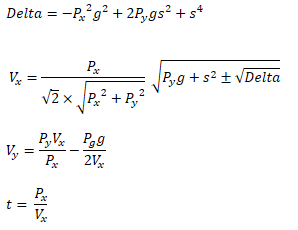In one of the prototypes I am working on recently, there are some enemy archers and I need them to be able to hit the player's character in movement. That is, knowing location and velocity of the target, the enemy archer's location and the speed of the arrow, I need to calculate a direction, or velocity for the archer to shoot the arrow. My assumption is that the arrow will subject to and only to gravity, while considering the velocity of the target when the projectile is launched. My code is an iterative method inspired by this post from Kain Shin for C++ in Unreal Engine, but I it can be easily adapted to another platform. Adding acceleration, although I haven't tried, should also work somehow using my method.

This post used KINSOL library to solve the problem, but it would eliminate the cross-platform potential of my game. The third method in this post doesn't work well if the upward/downward angle is very large. Considering the fact I already have a function working for non-moving targets, I decide to adapt from the second method in this post, which is an iterative way.

## Simplified case: assuming target does not move

It is easy to solve the equation if the target is not moving. I already had some code already working in DragonHunt (Thanks to Menglu!) to calculate direction to launch projectile, but it didn't take the movement of the target into consideration. (That is, the AI in the game needs to be smarter than that in DragonHunt XD)… Also, there is a function named SuggestProjectileVelocity in Unreal Engine that does exactly the same thing (C++, Blueprint)

I have shooter's position Po, position of the target Pt, gravity G. I have a downward axis in the direction of G:

Iy = normalized(G)

And I can calculate a front axis, and the direction of which is

Ix = normalized(cross(G, cross(Pt-Po, G))).

Not that it is possible the target is directly above or below the shooter, and we need to consider the special case - but I'll assume that's not happening for now.

And we can now do the whole calculation in the 2D space. I have target's relative position in Ix and Iy as

Px = dot(Ix, Pt-Po)
Py = dot(Iy, Pt-Po)
g = |G|

And what we need is to solve for the desired initial velocity of projectile in the space of Ix and Iy: Vx and Vy, in the following system:

• Vx^2 + Vy^2 = s^2 (S is initial speed)
• Vx * t = Px
• Vy * t + g * t^2 / 2 = Py
• Vx > 0
• t > 0
• (And Px, g, s should already be greater than zero if the inputs are correct)

We can have the following result:Finally we can calculate the velocity or direction of projectile we need to shoot in by

V = Vx * Ix + Vy * Iy

Note that there are two possible solutions. I would prefer the visual of higher arc of arrow in my game, so I'll just positive sqrt(Delta) in my case. We can prefer negative sqrt(Delta), to favor a shorter path if there is one.

If Delta is smaller than zero, the target is too far forward; if the things in the outer sqrt is smaller than zero, the target is too far upward. That's why I prefer my function than Unreal Engine's SuggestProjectileVelocity(), because I can alter the result (in my case Delta to zero if smaller than zero in order to try to shoot as long as the archer could - which just looks good) - and to calculate an "impact time" even if the target is far away, which would be important in the iterative method in order to hit a moving target, which can be initially out of archer's reach but moving towards the archer.

## Now the target has a velocity

If the target at P0 has a velocity Vt, we can call the function we have written above, and to calculate a projectile velocity V0 and impact time t0. After that, we can get calculate a new position of the target by P1 = P0 + Vt * t0.

Then, we use P1 as the new target position to calculate a new projectile velocity and V1 and travel time t1, which can be better than V0 and t0. And we have new arbitrary target position P2 = P0 + Vt * t1.

So, iteratively, we can calculate V[i] and t[i] from P[i] = P0 + Vt * t[i-1] . If target's velocity is not large, it should converge. And after some iterations (in my case 8), the initial velocity and direction of V[i] should hit the target. We can also introduce some interpolation to help convergence:

• P[i] = P0 + Vt * lerp(t[i-2],t[i-1], alpha) .
• V[i], t[i] = projectileVelocityForStaticTargetAt(P[i])

I used alpha = 0.8 and it reduceed the iterations needed to 5 to get a stable hit in my case.

And this is the code in Unreal C++ for the iteration:

```FVector UHelperLibrary::CalculateProjectileDirectionForMovingTarget(FVector Target, FVector TargetVelocity, FVector Origin, FVector Gravity, float Speed, bool & bWillHit, float & Time, int Iterations, float EpsilonTime)
{
if (TargetVelocity.IsNearlyZero())
{
// To hit a static target. You can also use SuggestProjectileVelocity;
return CalculateProjectileDirectionHelper(Target, Origin, Gravity, Speed, &bWillHit, &Time);
}

FVector PrevDirection;
float PrevTime = 0.0f;
float NewTime = 0.0f;
bool bHit = false;
constexpr float Alpha = 0.8f;

for (auto i = 0; i < Iterations; i++)
{
PrevTime = FMath::Lerp(PrevTime, NewTime, Alpha);
// Or use SuggestProjectileVelocity;
PrevDirection = CalculateProjectileDirectionHelper(Target + TargetVelocity * PrevTime, Origin, Gravity, Speed, &bHit, &NewTime);
if (NewTime == InfFloat)
{
bHit = false;
break;
}
if (FMath::IsNearlyEqual(NewTime, PrevTime, EpsilonTime))
{
break;
}
}

Time = NewTime;
bWillHit = bHit;
return PrevDirection;
}
```